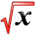# Marginal Cost/Profit/RevenueThis article is part of the MathHelp Tutoring Wiki

Definitions: Marginal cost MC(x)=C'(x)=d/dx C(x) Marginal revenue MR(x)=R'(x)=d/dx R(x) Marginal profit MP(x)=P'(x)=d/dx P(x) The marginal cost is the increasing in the cost of producing one additional unit. Note:

Since P(x)=R(x)-C(x)

d/dx P(x)=d/dxR(x)+ d/dx(-C(x))=d/dx*R(x)-d/dx*C(x)

Example1:

Question: A clothing store estimates that it has a fixed cost of \$8000 per month, and a cost of \$1.50 per shirt. It also estimates that it can sell a shirt at \$79.50 each but that this price decreased by \$0.50. For every shirt sold. Find the cost, revenue and profit function along with their marginals.

If x units are produced and sold per month.

C(x)= 8000+1.5x

The revenue,

P(x)= x(80-0.5)

P(x)=R(x)-C(x)=x*(80-0.5)-(8000+1.5)

=-0.5x2+78.5x-8000

Marginals,

MC(x)=d/dx C(x)=d/dx (8000+3x/2)

=3/2 d/dx x= 3/2

MR(x)=d/dx R(x)= d/dx (80-0.5x)x

=80-x

MP(x)= MR(x)-MC(x)=80-x-3/2=157/2-x

Marginal cost help you decide how to change production to increase the revenue. If marginal profit is possible. If marginal profit is positive, Then increasing production is beneficial, and visa versa.